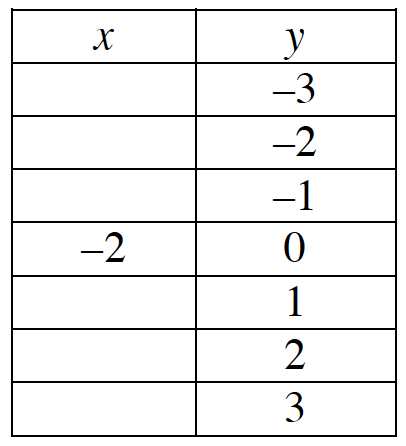### Home > PC3 > Chapter 5 > Lesson 5.2.5 > Problem5-116

5-116.

Graph $f(x)=\log_5(2+x)$ and state the domain and range.  Homework Help ✎

Rewrite the equation in exponential form.
$5^y=2+x$

Solve for $x$.
$5^y−2=x$

Make a table for the function. Choose values for $y$ and evaluate for $x$.Graph the function using the points in the table.